Analysis Of Magnetic Field Effect On Ferromagnetic Spheres Embedded In Elastomer Pattern

HOME / Applications / Analysis Of Magnetic Field Effect On Ferromagnetic Spheres Embedded In Elastomer Pattern
WEBINAR
Simultaneous Wireless Information and Power Transfer
Thursday, January 27, 2022
Time
SESSION 1
SESSION 2
CET (GMT +1)
03:00 PM
08:00 PM
EST (GMT -4)
09:00 AM
02:00 PM
Used Tools:General introduction

Magnetorheological elastomers (MREs) are a class of solids that consist of polymeric matrix with embedded micro- or nano-sized ferromagnetic particles such as carbonyl iron. As a result of this composite microstructure, the mechanical properties of these materials can be controlled by the application of magnetic field.

MREs are typically prepared by curing process for polymers. The polymeric material (e.g. silicone rubber) in its liquid state is mixed with iron powder and several other additives to enhance their mechanical properties. The entire mixture is then cured at high temperature.

Curing in the presence of a magnetic field causes the iron particles to arrange in chain like structures resulting in an anisotropic material. If magnetic field is not applied, then iron-particles are randomly distributed in the solid resulting in an isotropic material.

MREs hold promise in enabling simple variable stiffness devices. The pioneering application of MREs was presented in vehicle industry. A novel method and apparatus for varying the stiffness of a suspension bushing having an MRE disposed therein was invented. This allows for improved customer satisfaction by reducing dissatisfaction associated with braking events, such as shudder, which can result in increased noise, vibration and harshness.

It solves these problems by controlling relative displacements of longitudinal suspension member relative to a chassis member in a motor vehicle.

The control method is communicating a brake actuation signal from a brake actuator  to a suspension bushing stiffness and generating an electrical current in response thereto; communicating  the electrical current  to and an electrical coil operatively associated with the MRE, thereby generating a magnetic field so as to vary the stiffness characteristics of the MRE in a way to reduce an operator's perception of brake induced shudder.

Example of industrial application of MRE's

Vibration isolation consists of isolating vibration sources from the parts by installing vibration isolators between them.

An example of a vibration isolator is proposed by Liao et al . It consists of a tunable stiffness and damping vibration isolator using an MR elastomer.

The isolator is formed by the following parts: base, magnetic coils, magnetic conductor, shear plate, iron core, MR elastomer, voice coil motor and a mounting plate. Three coils were used to generate magnetic field for 4 layers of MR elastomers to provide tunable stiffness. The voice motor was used to generate force, which is proportional to the shear velocity. Under such a mechanism, tunable stiffness and damping are achieved.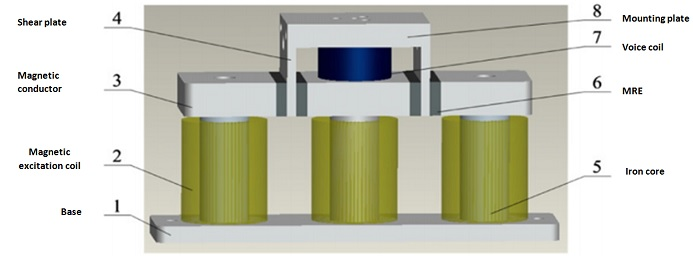Figure 1 - Schematic of the MR elastomer-based vibration isolator designed by Liao and al.

Description of the problem

The present model consists of a wound coil surrounding an elastomer pattern, this latter holds two ferromagnetic spheres. Attraction forces will be crated between the spheres once the coil is traversed by a constant electrical current.

The main focus is on the effect of the magnetic field on the  two ferromagnetic spherical bodies. The whole analytical solution concerning the knowledge of attraction of the spheres is usually simplified by the assumption of one concentrated force acting in the gravity center of the body. Such an approach allows one to obtain only approximated results, however, the actual numerical model  makes possible the determination of forces in each point of the discrete bodies.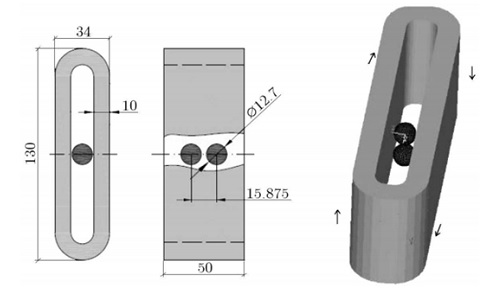Figure 2 - Dimensions of the solenoid and spheres.

Multiphysics simulation using EMS for SolidWorks

EMS ensures a multi-physical simulation by the capability of coupling between magneto-mechanical fields.

In this example, it helps to understand influence of an applied constant magnetic field on disposition of two ferromagnetic bodies. This coupling is sequential. The main goal is to outline the magnetic forces exerted on the ferromagnetic spheres. The resultant displacement and  Von Mises stress are calculated based on the knowledge of the magnetic forces.

Materials

Below in table 1 are the properties of the used materials.

Table 1. Material properties assigned to the model parts.

 Relative Permeability Electrical Conductivity  (Mho/m) Elastic modulus  (Pa) Poisson's  ratio Copper 1 5.998e+07 Not required Not required Steel 10e+04 Not required 2e+11 0.3 Elastomer 0.99999 0 12e+04 0.45 Air 1 0 Not required Not required

Electromagnetic inputs

A wound coil is defined as  the current source of the problem. Properties of it is given in table 2 below.

Table 2 - The coil properties.

 Number of turns Wire diameter (mm) Current amplitude (A) Wound Coil 1 1 0.91168568 mm 5000

Structural Inputs

Fixed constraint is applied on the following highlighted  faces of the elastomer box, shown in figure 3.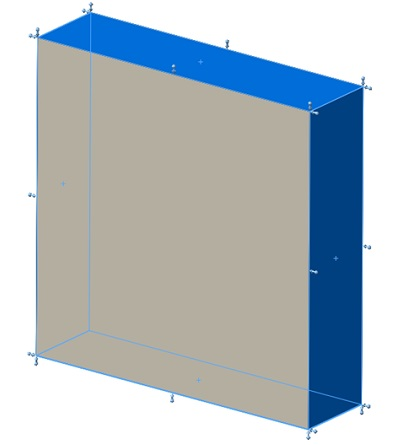Figure 3 - Fixed constraint applied on the model faces.

Meshing

The accuracy that should be obtained from any FEA model is strongly dependent on the finite element mesh used. The finite element mesh is used to subdivide the CAD model into smaller domains called elements, over which a set of equations are solved. EMS uses tetrahedral mesh element. You can choose to refine or coarsen the mesh, using EMS meshing features, depending on the nature of your analysis and on the geometry of the model.

The mesh used for the ferromagnetic spheres is given in figure 4. The mesh of the two spheres should be refined to get accurate magnetic force calculation and to guarantee enough elements for stress and deformation calculation. We should bear in mind that the main focus is on the changes of position of the spheres once traversed by a magnetic field.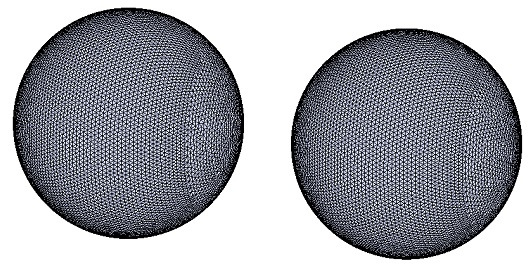Figure 4 - The steel spheres mesh.

Magneto-mechanical results

EMS offers the possibility of many types of plots. Below we can observe fringe plot of the magnetic flux density.

Magnetic Flux density plot

Figure 5 shows magnetic flux distribution in the elastomer pattern.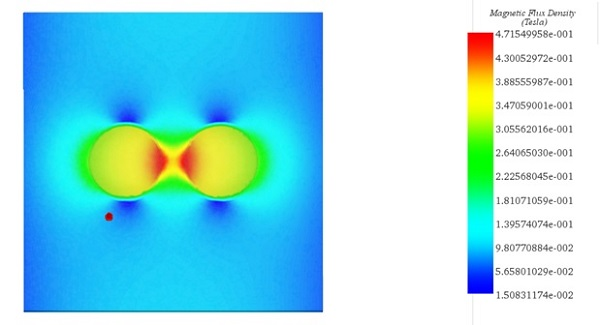Figure 5 - Section clipping plot of magnetic flux density in the elastomer pattern.

Magnetic Force density plot

Magnetic force is concentrated on the boundary of the ferromagnetic bodies. This is explained by the difference of permeability between  the two mediums (the nonmagnetic  elastomer and the ferromagnetic spheres).Those forces are called Maxwell forces or also reluctances forces, are concentrated at the interface of high magnetic reluctivity changes, e.g. between air and a ferromagnetic material. They are also responsible of the attraction or repulsion of two magnets facing each other.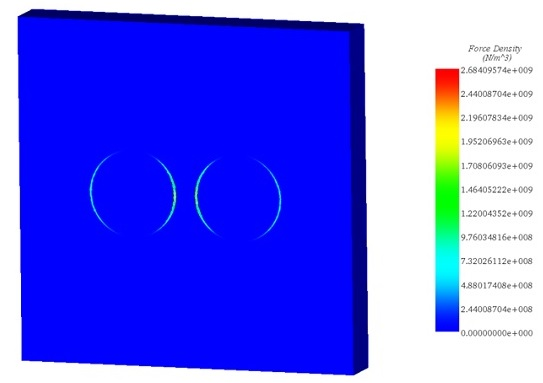Figure 6 - Magnetic force density in the plane passing through the gravity center of the spheres.

Displacement plot

Maximum deflection occurs in the elastomer pattern, as shown in figure 7.  Direction of the vector shows that the two ferromagnetic spheres attract each other under the effect of Maxwell forces . Maximum displacement is equal to 0.11mm.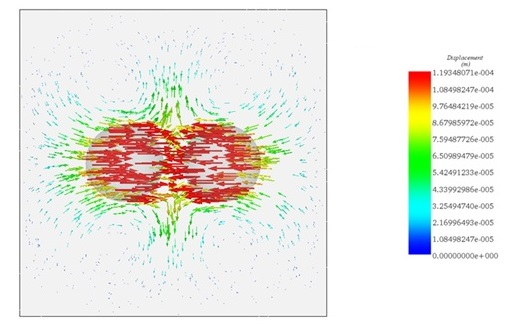Figure 7 - Vector plot of displacement in the plane passing through the gravity center of the spheres.

Stress plot

Von Mises stress plot in the plane passing through the spheres center of gravity is given in figure 8.  Stress is mostly uniform within the elastomer and is equal to about  1.4 e4 N/m^2 (Pa).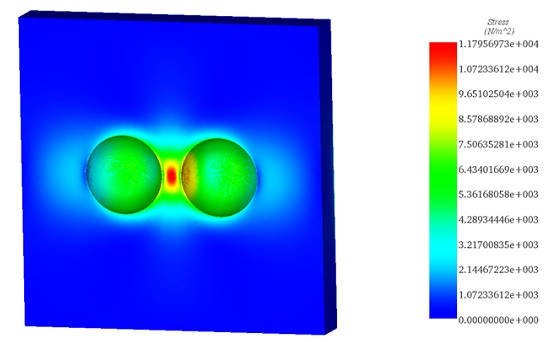Figure 8 - Von Mises stress in the elastomer (section clipping is done by the plane passing through the gravity center of the spheres).

Conclusion

Magneto-structural analysis of two ferromagnetic bodies is done inside EMS for SolidWorks. Magnetic and mechanical output quantities are in excellent agreement with experimental and numerical results given in .

Plots of magnetic field and magnetic flux density show that the magnetic field distribution in a nonmagnetic medium (the elastomer pattern in our example) is strongly influenced by the shape of the ferromagnetic bodies.

It's worth noting that when a higher current is applied on the coil, the spheres will collide.

References

: A. Boczkowska, L. Czechowski, M. Jaroniek and T. Niezgoda.2010. Analysis of magnetic field effect on ferromagnetic spheres embedded in elastomer. Journal of theoretical and applied mechanics. Volume 48, issue 3, pp.659-676: Warsaw.

: https://en.wikipedia.org/wiki/Magnetorheological_elastomer

:Yancheng Li, Jianchun Li, Weihua Li and Haiping Du. Australia 2014. A state of the art review on Magnetorheological elastomer devices. Smart materials and structures. 23(12), pp.1-24.